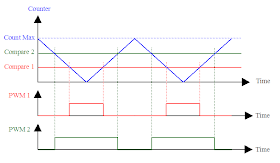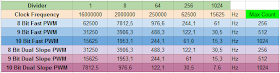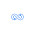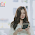## Monday, December 22, 2014

### Fast PWM on Arduino Leonardo

Today I will talk about PWM generation.
The Arduino boards provide pseudo analog outputs using the analogWrite( ) function. This function is not available on all pins, only the ones marked with the ~ symbol. The Analog Write function doesn't provide a real analog output but a PWM signal instead.Arduino Leonardo Board
A PWM (Pulse Width Modulation) signal is a pulsed binary signal. As it is binary it can only have two output states "HIGH" and "LOW". The analog information is not on the signal levels but on the width of the generated pulses.We define Pulse Width as the width of the HIGH pulses and Duty Cycle, represented with a lower case delta letter, as the fraction of the Pulse Width to the total period T of the signal. The frequency of the PWM signal is defined as the inverse of the period.PWM Signal

The mean value of the PWM signal depends on the Duty Cycle and the voltage values associated to the HIGH and LOW levels:
If the LOW level is zero, the the mean value of the PWM signal is:

That way, the mean value of the signal is proportional to the Duty Cycle.

Arduino analogWrite( ) function maps a 0 to 255 input value to a 0% to 100% Duty Cycle. As the HIGH level is about 5V and the LOW level is near to zero, the mean value of the signal generated using  analogWrite(pin,x) is:
If the device connected to the pin has lower bandwidth than the frequency of the PWM signal, it will work as low pass to the signal an only will see the mean value. Arduino Leonardo PWM pins use frequencies of  488Hz or 976Hz. When you use analogWrite on a LED, as LEDS have usually higher than 1kHz bandwidth, the LED will turn full ON and OFF as indicated on the PWM signal. Our eye, however, has a bandwidth (fusion frequency) up to about 100 Hz depending on the ambient ligh levels. As the LED pulses at a higher frequency than the eye bandwidth, we only see the mean value and seems that the LED lights at intermediate levels between ON and OFF.
The same applies if we use a PWM signal to drive a motor. Normal DC motors, due to their inertia, have bandwidths near or below the Arduino PWM frequency, so the motor works as if a constant variable voltage where applied. In fact, PWM operation at low frequency can make the motor work better at low speeds.

All in all, the 500Hz to 1kHz PWM frequency in stock Arduino is adequate to drive motors. If we want, however, to generate an audio signal, the analogWrite function does not work.The hearing bandwidth in humans is arround 20kHz, much higher than the Arduino PWM frequency. Typical speakers are usually designed inside the human hearing bandwith so applying a PWM signal to them will produce an audible tone at the PWM frequency.

One of the utilities to generate a high frequency PWM signal is going beyond the speaker and hearing bandwidths so that we can use an PWM signal to generate an audio signal.

### Stock PWM on Arduino Leonardo

First we start explaining how the PWM is implementated on the Arduino Leonardo board. In most Arduino boards the PWM signals are generated using timers. Timer peripheras provide hardware PWM generation so that the CPU don't need to use any of it's execution resources to generate the signals. Each timer has a limited number of PWM signals than can be generated by hardware.

The Arduino Leonardo, as the Micro and Esplora use the ATmega42u4 MCU. It includes four timers: Timer 0, Timer 1, Timer 3 and Timer 4. Timers 1 and 3 are equal, but the rest of timers are quite different. Other Arduino Boards make use of other timers provided by the MCUs they use.

The following table shows the timers usage to generate PWM on the Arduino Leonardo board. You can see it and more information on my Arduino Leonardo spreadsheet.PWM Timers in Arduino Leonardo
Arduino uses the timers for other functionalities. For instance, Timer 0 is also used to record the pass of time as is needed for the functions millis( ), micros( ), delay( ) and delayMicroseconds( ). You don't wanna mess with Timer 0 operation as it will disturb all timing functions.
Some Arduino libraries make also use of timers. The Servo library uses Timer 1 on the Leonardo while the MsTimer2 library uses Timer 4. You should know that using any library that needs a timer will affect any PWM pin associated to that timer.

There are several two basic ways to create a PWM signal using a timer.

### Single Slope PWM

The timers are typically based on a Counter. The counter uses a clock input and, at each active clock edge, the counter changes state. One typical mode of operation for a timer involves increasing the counter at clock each clock edge until it reaches a maximum value. Once this value is reached, the counter returns to zero and the process repeats.That gives a sawtooth waveform on the counter values as is depicted in the figure below. One full cycle of the sawtooth will need Count Max + 1 clock cycles.Single slope PWM

Timer peripherals usually include several capture/compare registers. In order to create a PWM signal, a compare register is linked to a hardware output of the MCU in a way that makes this output high if the counter is below or equal to the compare register value. That will give, for each compare register, a PWM signal whose duty cycle depends on the compare value.
All PWM signals generated by the same timer, will have the same frequency as the start of the generated pulses will allways be at the falling edge of the sawtooth signal.
If we have several PWM signals regenerated with different compare registers at the same timer, all of them will have the rising edge at the same times.

The Arduino analogWrite( ) functions operate the ATmega 32u4 Timer 0 at single slope. The 250kHz clock used is obtained dividing by 64 the 16MHz system clock. The final PWM frequency will the be 976Hz.
Timer 0 has two compare channels A and B associated to two compare registers OCR0A and OCR0B. The two channels are linked to two hardware outputs OC0A and OC0B that are used as Arduino pins numbers 11 and 3.

### Dual Slope PWM

Another way to generate a PWM signal configures the counter to increase at each clock edge, and when it reaches the maximum value, start decreasing one number each clock cycle until it reaches zero and the patter repeats again. That will give a triangle waveform instead of the sawtooth one.Dual Slope PWM
We can also configure a capture register so that a generated output signal is high when the counter is below this register. This will generate a PWM signal as in the single slope case. The difference is that, if we have several compare registers, the PWM signal generated with each one will be synchronized at the center of the generated pulses.
This is handy in some kinds of motor control but the details are beyond this article.
In any case, the PWM frequency will be the same for all compare channels and will also be half of the value we would obtain if we used a Single Slope counter.

The Arduino analogWrite( ) functions operate the ATmega 32u4 MCU Timers 1, 3 and 4 in Dual Slope mode. A possible reason why Timer 0 is not operated also in Dual Slope can probably be due to the fact that Timer 0 is used to record the pass of time and, this use, is difficult to be implemented on a dual slope timer. Working on dual slope, all PWM pins associated to those pins use half the Timer 0 frequency working at 488Hz.
Timer 1 includes three compare channels A, B and C associated to compare registers OCR1A, OCR1B, OCR1C linked to hardware outputs OC1A, OC1B and OC1C. The Arduino software only uses the channels A and B that are related to Arduino pins 9 and 10. Channel C output OC1C is associated to the same 11 Arduino Pin driven by Timer 0. For whatever reason Arduino designers preferred to drive pin 11 using Timer 0 at single slope than use Timer 1 at dual slope.
Timer 3 only includes one hardware output OC3A at Arduino pin 5, associated to the compare register OCR3A.
Timer 4 is a special timer, but respect to PWM, it operates as Timers 1 and 3 in dual slope mode. It includes three hardware outputs OC4A, OC4B and OC4D associated to registers OCR4A, OCR4B and OCR4D. Only channels A and D are used in stock Arduino for pins 13 and 6. Channel B is associated to the same pin driven by OC1B so Timer 1, instead of Timer 4, is used for this pin.
All of that gives the table of the 7 PWM enabled Arduino Pins.

### Fast PWM on Timer 1

If the maximum 976 Hz that stock Arduino analogWrite is not enough, we need to develop our own PWM functionality. Timer 1 is a good candidate as it has three available compare channels and don't mess with Arduino delay functions. You should take care if you use the Servo library because it also uses Timer 1.

Timer 1 is based arround a 16 bit counter. That means that it can count from 0 up to 65535 before overflowing. The timer has several modes of operations that include 12 PWM modes. The fastest PWM mode available is single slope 8 bits counting between 0 and 255. As single slope is faster that dual slope, it is also called Fast PWM mode.
You can also have 9 bits and 10 bits PWM modes with 511 and 1023 terminal counts that can operate on single and dual slope modes. Three bit modes 8, 9 and 10 for single and dual slope PWM gives a total of 6 PWM modes. The other 6 additional PWM modes use programmable terminal counts that can be any 16 bit value and is not restricted to 255, 511 or 1023.

The timer gets its clock from the global MCU prescaler that provides five frequencies from the system clock. The divide ratios are 1, 8, 64, 256 and 1024. That gives, using the 16MHz Arduino Leonardo system clock, the fiveclock and single slope frequencies shown below for standard 8, 9 and 19 bit modes:Prescaler and PWM options
Using dual slope the maximum frequency will be half. We see that the maximum available PWM frequency on Timer 1 is 62.5kHz. That's enough to generate some sort of audio signal as it is beyond the audible range of frequencies.

In order to configure the timer we must program the Timer 1 registers TCCR1A and TCCR1B.Bits 0, 1 and 2 of TCCR1B (CS10, CS11 and CS12) configure the clock options acording to the following table.Bit 1 of TCCR1A (WGM11) and bits 3 and 4 of TCCR1B configure the waveform for the timer acording to the table:

Modes  1, 2, 3, 5, 6 and 7 correspond to the 6 standard PWM modes.

Once the timer is configured on one PWM mode, each of the three compare channels A, B and C can be enabled to generate a PWM signal. To do that, the COM1x0 an COM1x1 bits on register TCCR1A associated to a particular x channel (A, B, C) need to be configured according to the table:

The PWM value for each channel should be programmed in each channel compare register OCR1A, OCR1B and OCR1C.

All the above tables habe been taken from the ATmega32u4 datasheet.

Configuring the TCCR1A bits is not enough to generate the PWM signal at the output pins. We also need to configure them in output mode. From the ATmega32u4 we can see that the timer 1 outputs OC1A, OC1B and OC1C  are associated to port lines PB5, PB6 and PB7 (Arduino Pins 9, 10 and 11). We need to set those pins in output mode using the Port B Data Direction Register DDRB.

### Fast PWM on Timer 4

Using Timers 0, 1 or 3  we can have up to 62,5kHz PWM signals, but the maximum possible PWM frequency is only available on Timer 4.
Timer 4 is a 10 bit timer that can operate at very fast speed due to its clock source options.
The ATmega32u4 MCU includes an USB peripheral. This peripheral needs a 48MHz clock frequency that goes beyond the maximum 16MHz system clock. In order to generate the USB frequency the MCU incorporates an internal PLL. In the Arduino Leonardo the PLL takes as input the 16MHz system clock and multiplies it by 6 to generate a 96MHz output frequency.
You don't want to mess with the PLL configuration on the Arduino Leonarda as it will break the USB communications. The only need you need to know about the PLL is that its output can source the Timer 4 peripheral.

The PLL register PLLFRQ includes among other things, two bits PLLTM0 and PLLTM1 that determine the input clock to Timer 4.

There are four options, don't use the PLL to source the timer (it will be sourced by system clock) or to use the PLL output divided by 1, 1.5 or 2. That will give 96MHz, 64MHz or 48MHz clock frequencies.

At the time of this writting the above table was missing from the official ATmega32u4 current datasheet. I needed to find an older datasheet to obtain the full PLL configuration register contents.

Timer 4 has an additional divider configured with bits CS40 to CS43 of the TCCR4B configuration register.

The available options are:

That way, using the PLL and Timer 4 dividers you can have input frequencies betwen 96MHz and 5859Hz.

In a similar way than Timer 1, Timer 4 must select a waveform operation mode using bits WGM40 and WGM41 of the TCCR4D register.

The available options are:

Mode 00 is Single Slope while mode 01 is Dual Slope.

Observe that the PWM signal has a maximum count value defined in OCR4C register. As compare channel C register is used for the terminal count, there is no independent channel C PWM output.

PWM6 is an special PWM operation mode that uses all three available channels A, B and D to drive a motor. The details, that are beyond the scope of this document, can be found on the MCU datasheet.

After configuring the clock input, each channel x = A, B and D of Timer 4, can be configured with its own bits COM4x0, COM4x1 and PWM4x at registers TCCR4A and TCCR4C.
As we have explained channel C, used as terminal count, has no output unit so it cannot be used to generate PWM.
To operate one x channel in PWM mode you need to set to "1" the  corresponding PWM4x bit. After that, the COM4x0 and COM4x1 determine the mode of PWM operation. In the case of channel A in fast PWM mode we can choose:

Normal PWM operation corresponds to mode 10. Mode 11 will give a complementary output whereas mode 01 gives two complementary PWM outputs at different pins. The complementary signals can have some deadband between them so that one signal, and its complement are never active at the same time.
Similar tables can be found for channels B and D.

### Fast PWM Test Code

I have put together all the above methods to generate PWM in a FastPWM Arduino sketch.

The sketch includes the needed code to operate 5 pins associated to Timers 1 and 4 in fast PWM modes.

Timer 1 Code

The code associated to timer 1 includes 4 functions and several defines. It can uses all three Timer 1 compare channels to generate PWM signals at Arduino pins 9, 10 and 11.

The pwm91011configure function must be called previously to the call of any other function associated to this timer. It configures the timer to operate in single slope fast PWM mode and sets the prescaler to the mode indicated on the function argument.
The five possible modes are  PWM62k,  PWM8k, PWM1k, PWM244 and PWM61 and are associated to the four available frequencies in single slope 8 bit PWM modes.

Functions pwmSet9, pwmSet10 and pwmSet11 configure the Timer 1 channels associated to pins 9, 10 and 11 to work in PWM mode and set the given PWM value (0 to 255).

After one of the three above functions is called, the PWM value can be fast changed using a direct access to the corresponding compare register. To ease the access, three definitions PWM9, PWM10 and PWM11 are associated to compare registers OCR1A, OCR1B and OCR1C.

Timer 4 Code
The code associated to timer 4 includes 3 functions and several defines also. It uses two of the three available compare channels A and D associted to Arduino pins 13 and 6. It doesn't use channel B because its Arduino pin 10 conflicts with the Timer 1 channel B previously used.
The pwm613configure function is similar to the one defined for Timer 1. It sets the PLL and PWM modes to properly configure Timer 4 in high speed mode from the 48MHz PLL tap and sets the terminal count in OCR4C to 8 bits (255).
The input argument sets one of seven available prescaler frequencies for a 48MHz timer 4 clock input: PWM187k (187500 Hz), PWM94k  (93750 Hz), PWM47k  (46875 Hz), PWM23k  (23437 Hz), PWM12k  (11719 Hz), PWM6k   (5859 Hz) and  PWM3k  (2930 Hz). If you remenber the Timer4 options there where 15 clock prescaler options. The lower frequency ones are not implemented in the defines as they provide no advantage over stock analogWrite functionality.

Functions pwmSet13 and pwmSet6 configure the Timer 4 channels associated to pins 13, 6 to work in PWM mode and set the given PWM value (0 to 255).
In a similar way than timer 1, after one of the two above functions is called, the PWM value can be fast changed using a direct access to the corresponding compare register. Two definitions PWM13, and PWM6 are associated to compare registers OCR4A and OCR4D. An aditional PWM6_13_MAX definition has been added to access the OCR4C register that sets the PWM terminal count that is, by default, set to 255 (8 bits).

Setup and Loop Code

To check all the above functions Timer 1 is configured in setup to generate 62,5kHz PWM signals.  Timer 4 is configured for 187kHz. 4 signals are generated. At pins 11 and 13 11% and 75% fixed PWM values are set.  For pins 6 and 9, a variable 0% to 100% PWM value is programmed inside the loop function.

### Generated Waveforms

The following figure shows the generated waveforms captured with the Logic Analizer of the Analog Discovery scope.

Timer 1 signals at pins 9 and 11 have the same risign edge as they are in single ramp PWM mode. The same applies to signals at pins 6 and 13 that depend on Timer 4. Signals at different timers are at different frequency so the rising edges don't usually coincide for both timer signals.

That's all for now. Timers are very usefull peripherals. PWM is only one of the timer applications. In the future I plan to talk also about generation of periodic events.

### Code on Github (11/02/2018)

The code is now on Github:

https://github.com/R6500/Leonardo/blob/master/FastPWM.ino

1.Thanks for that great tutorial.
Is it possible to use Pin 5 with fast PWM, too? Instead of Pin 13? And how?
OCR4A=value; // Set PWM value
DDRC|=1<<6; // Set Output Mode C6
... ??
Wolfgang

2.This comment has been removed by a blog administrator.

3.I solved it:
OCR4A = 255 - value; // Set the negativ PWM value
DDRC |= 1 << 6; // Set Output Mode C6
TCCR4A = 0x42; // Activate the complementary channel A
Thanx
Wolfgang

4.Hi and thank you.

Can you explain how do you use D11 with timer 1 with D9 and D10 ?

1.D11 is associated to Timer0 Channel A or Timer 1 Channel C
Arduino code uses the first option
My code uses the second option in function "void pwmSet11(int value)"

5.6.Hi! great tutorial!
I am trying to use this in conjunction with the following 10bit pwm example:
http://blog.saikoled.com/post/43165849837/secret-konami-cheat-code-to-high-resolution-pwm-on
In order to have high speed 10 bit pwm on pin D6.

I am writing the top two bits to TC4H like this:
TC4H = value >> 8;
OCR4D = value;

But i cant seem to expand the OCR4C compare value beyond 255, it seems to store maximum one byte. If i set it to 256 it behaves as though i had set it to 1.

Any idea what i'm missing?

Cheers

7.Very very interesting explanation. Will have to read it a few times but time is on my side, thanx.

8.Hello thanks for the explanation, but what if i want to produce an other frequency?

How do i do to produce a square wave at 40Khz by the ... pin 9 for example???

9.When using this on a DC motor and uploading, the motor runs fine. If you unplug the USB and power up later, there is an audible noise from the motor due to the PWM, it seems the frequency becomes lower after the USB is unplugged after uploading. I don't have a scope to hand to measure what freq. is being generated.

Any ideas?

1.I don't recommend using fast PWM on a motor
For motors, stock 500 Hz or 1 kHz on normal Arduino PWM is ok
Using on motors a frequency above the minimum needed will decrease the driver eficiency
And, of course, all kind of noises due to to mechanical interactions with the motor elements can be produced

10.Fantastic so much needed info in one short post! Thanks.
That spreadsheet is perfect too! Wow thanks.

I had to re read a few bits as they didnt make sense - I think they are typos:

"Timer 3 only includes one hardware output OC1A at Arduino pin 5, associated to the compare register OCR3A."

"Timer 3 only includes one hardware output OC3A at Arduino pin 5, associated to the compare register OCR3A."
&

"Timer 4 Code
The code associated to timer 1 " Should this not read "The code associated to timer 4 "

1.Yes, they are typos
I usually write too fast and they slip in
Thanks

11.It's truly awesome and I like to read this type of impressing article. So I like to thank you for sharing a great article. Ethernet cords

12.Надявам се, че имате по-добри статии. Поздрави. Твърде щастлив

Máy massage chân

Chậu ngâm chân

Bồn ngâm massage chân

13.Hi Vicente: I'm developing a project to measure mass described on a page at http://www.skillbank.co.uk/arduino/mass.htm. I will need to use fast PWM to generate the waveform. Is it OK if I link to your page here?
Thanks, John

14.Nice Blog, Thanks for share an amazing blog web portal development Keep Posting

15.Thank you so much. I am happy if the word is spread.FANUC PARTS
Much obliged for the insight.

16.This comment has been removed by the author.

17.Can we use timer0 if not using any timing related functions such as delay() in our code? How would we implement this?

18.Thank you so much!! Excellent article!